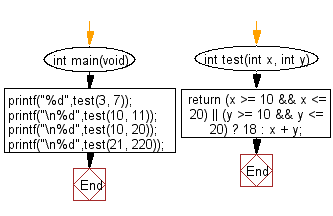﻿ C : Add two integer, Check if one of them is between 10..20# C Exercises: Compute the sum of the two specified integers. If one of the given integer value is in the range 10..20 inclusive return 18

## C-programming basic algorithm: Exercise-22 with Solution

Write a C program to compute the sum of the two given integers. If one of the given integer value is in the range 10..20 inclusive return 18.

C Code:

``````#include <stdio.h>
#include <stdlib.h>

int main(void){
printf("%d",test(3, 7));
printf("\n%d",test(10, 11));
printf("\n%d",test(10, 20));
printf("\n%d",test(21, 220));
}
int test(int x, int y)
{
return (x >= 10 && x <= 20) || (y >= 10 && y <= 20) ? 18 : x + y;
}
``````

Sample Output:

```10
18
18
241
```

Pictorial Presentation:Flowchart:C Programming Code Editor:

What is the difficulty level of this exercise?

Test your Programming skills with w3resource's quiz.

﻿

## C Programming: Tips of the Day

Why doesn't a+++++b work?

printf("%d",a+++++b); is interpreted as (a++)++ + b according to the Maximal Munch Rule!.

++ (postfix) doesn't evaluate to an lvalue but it requires its operand to be an lvalue.

! 6.4/4 says the next preprocessing token is the longest sequence of characters that could constitute a preprocessing token"

Ref : https://bit.ly/3fdldUT